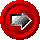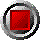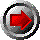Level Principal Swaptions (Black-76 model)Level Principal Swaptions (Black-76 model)

The functions in this category compute price, risk management statistics ("Greeks") and implied volatilities for European swaptions using the Black-76 model. The functions receive array arguments to define the zero curve (curve dates and zero rates or discount factors). The Zero Curve Methodology section discusses the form of these arrays and relevant restrictions in greater detail.

PayerSwaption_Black(argument list…)

These functions value European swaptions on level principal structures. If needed, short first stub periods are generated. The calling functions allow the user to choose from six different calculations, indexed by an alphabetic Output_Type switch:

 P option price D delta (derivative with respect to forward yield) G gamma (second derivative with respect to forward yield) V vega (derivative with respect to volatility, 1% change) T theta (time decay over next day) I implied yield volatility

An Output_Type may be entered in either upper or lower case, and the user many substitute the entire word for the first letter if so desired (only the first letter is scanned). Delta and gamma are returned in terms of swaps (i.e. an at the money swaption would have a delta of approximately +/-0.5). The price, vega, and theta are in terms of percentage of the notional of the swap.

The functions use the following arguments:

 Argument Description Restrictions Output_Type output returned by function P, D, G, V, T or I (see above) Valuation_Date valuation date (e.g. today) valid Excel date number Swaption_Maturity_Date expiry date of the swaption valid Excel date number >= valuation date Swap_Start_Date start date of swap valid Excel date numbers >= Swaption_Maturity_Date Term_in_Months term of the underlying swap in months > 0 (integer) Swap_freq number of fixed side payments per year 1, 2, 4, or 12 Year_Basis year basis used in determining payments 360 or 365 Fwd_Par_Swap_Rate current fair fixed swap rate in decimal form (e.g. six percent entered as 0.06) [see FwdParSwapRate function] > 0 Strike_Rate strike rate of the swaption in decimal form > 0 Zero_Dates array of zero coupon curve dates strictly ascending order The first date of this array must be Valuation_Date Zero_Rates array of continuously compounded riskless rates in decimal form (e.g. six percent entered as 0.06) corresponding to Zero_Dates > 0 Yield_Vol or Opt_Price underlying swap rate volatility in decimal form, or market price of the swaption (when Output_Type = I) > 0 Holidays array of holiday dates valid Excel date numbers strictly ascending order Convention date convention used in generating swap dates 0 = no adjustment 1 = Following business day 2 = Modified following 3 = Previous

Swaption_Black(argument list…)

This function is identical to those above, except that there is an extra argument (at the beginning of the argument list) which permits the user to choose between three different types of swaptions using a Swaption_Type alphabetic switch: